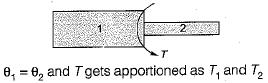Courses

# Test: Torsion of Shafts - 1

## 10 Questions MCQ Test Topicwise Question Bank for Mechanical Engineering | Test: Torsion of Shafts - 1

Description
This mock test of Test: Torsion of Shafts - 1 for Mechanical Engineering helps you for every Mechanical Engineering entrance exam. This contains 10 Multiple Choice Questions for Mechanical Engineering Test: Torsion of Shafts - 1 (mcq) to study with solutions a complete question bank. The solved questions answers in this Test: Torsion of Shafts - 1 quiz give you a good mix of easy questions and tough questions. Mechanical Engineering students definitely take this Test: Torsion of Shafts - 1 exercise for a better result in the exam. You can find other Test: Torsion of Shafts - 1 extra questions, long questions & short questions for Mechanical Engineering on EduRev as well by searching above.
QUESTION: 1

Solution:
QUESTION: 2

### The following torsional terms is analogous to bending terms except

Solution: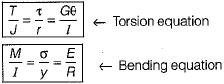QUESTION: 3

### The safe twisting moment for a compound shaft is equal to the

Solution:

If we take maximum calculated value, shear stress developed in other shaft of compound shaft would exceed the permissible design stress. This will fail the design, so minimum calculated value is taken as safe twisting moment.

QUESTION: 4

The ratio of maximum shear stress to maximum normal stress at any point in a solid circular shaft is

Solution: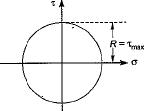(Mohr’s circle for shaft subjected to torsion)
Radius of circle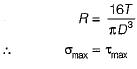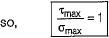QUESTION: 5

Strain energy stored in a solid circular shaft is proportional to

Solution: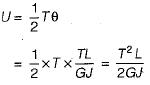QUESTION: 6

The value of shear stress which is induced in the shaft due to the applied couple varies

Solution: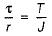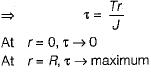QUESTION: 7

Shear stress induced in a shaft subjected to tension will be

Solution:
QUESTION: 8

Two solid shafts are made of same material and have their diameters D and D/2. The ratio of strength of bigger shaft to smaller one in torsion is

Solution:

Strength means torque carrying capacity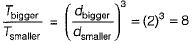QUESTION: 9

Compare the strengths of solid and hollow shafts both having outside diameter D and hollow shaft having inside diameter of D/2 in torsion. The ratio of strength of solid to hollow shafts in torsion will be

Solution: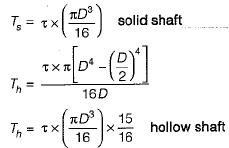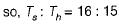QUESTION: 10

Torsion bars are in series

Solution: